Next:5.4.3 B-Trees: InsertionUp:5.4 B-TreesPrevious:5.4.1 Definition of B-Trees

## 5.4.2 Complexity of B-tree Operations

Let there be n records. If each leaf has b records on the average, we have,

average number of leaves = L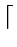n/b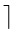Longest paths occur if the number of children at every stagem/2= l, say

Average number of nodes that are parents of leaves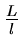Average number of nodes that are second level parents

=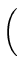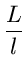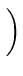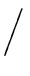l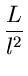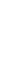If h is the level of the leaves, we have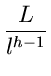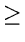1 or hloglL i.e., hlog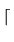m/2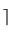n/bIf n = 106 records (1 million records), b = 10, and m = 100, we have

h3.9

eEL,CSA_Dept,IISc,Bangalore